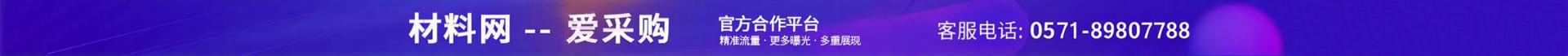•••e="1396" et="1">

me="36276">

e="1405" et="4">

e="6225" et="2">

e="1994" et="5">